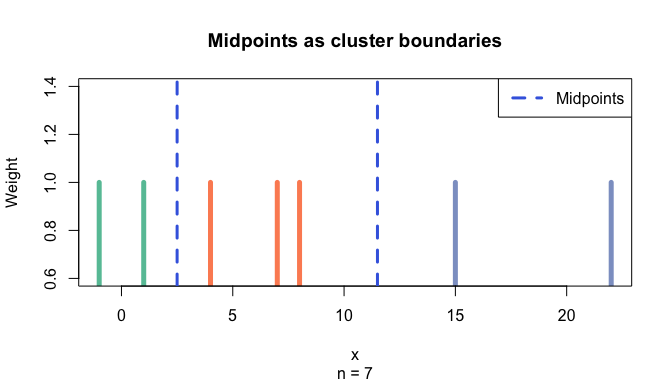# Tutorial: Optimal univariate clustering

#### Updated 2020-11-07

Here, we illustrate optimal univariate clustering function Ckmeans.1d.dp. It uses a given number of clusters $$k$$, or estimates $$k$$ if a range is provided. If $$k$$ is unspecified, a default range from 1 to 9 is used. It can also perform optimal weighted clustering when a non-negative weight vector is specified as input. Weighted clustering can be used to analyze signals such as time series data, spectral data, genetic or epigenetic events along a chromosome.

require(Ckmeans.1d.dp)
require(RColorBrewer)

## 1. Cluster univariate data given the number of clusters $$k$$

### Example 1.

Cluster data generated from a Gaussian mixture model of three components.

The number of clusters is provided.

x <- c(rnorm(50, sd=0.3), rnorm(50, mean=1, sd=0.3), rnorm(50, mean=2, sd=0.3))
k <- 3 # Divide x into 3 clusters
result <- Ckmeans.1d.dp(x, k)
colors <- brewer.pal(k, "Dark2")
plot(result, col.clusters = colors)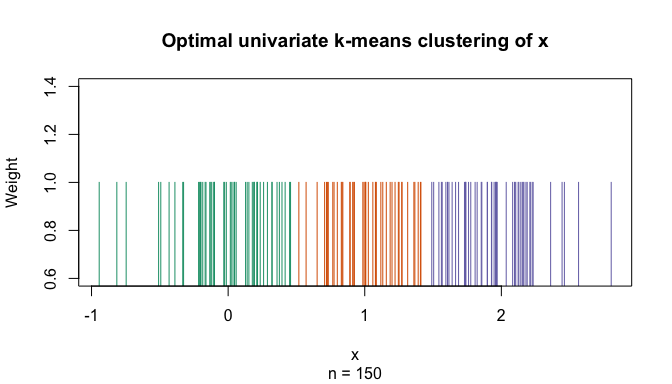plot(x, col=colors[result$cluster], pch=result$cluster, cex=1.5,
main="Optimal univariate clustering given k",
sub=paste("Number of clusters given:", k))
abline(h=result$centers, col=colors, lty="dashed", lwd=2) legend("bottomright", paste("Cluster", 1:k), col=colors, pch=1:k, cex=1, bty="n")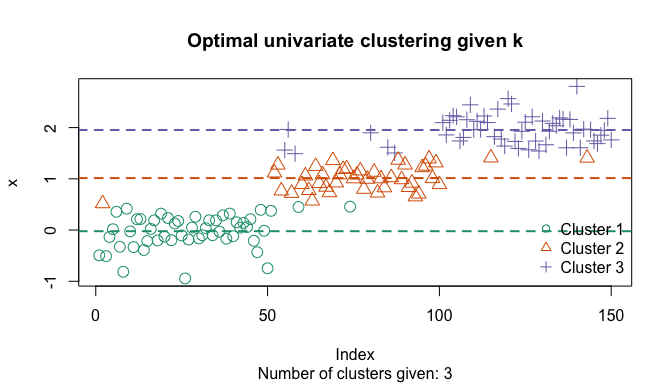## 2. Cluster univariate data with an estimated number of clusters $$k$$ ### Example 2. Cluster data generated from a Gaussian mixture model of three components. The number of clusters is determined by Bayesian information criterion: x <- c(rnorm(50, mean=-1, sd=0.3), rnorm(50, mean=1, sd=1), rnorm(50, mean=2, sd=0.4)) # Divide x into k clusters, k automatically selected (default: 1~9) result <- Ckmeans.1d.dp(x) k <- max(result$cluster)
colors <- brewer.pal(k, "Dark2")
plot(result, col.clusters = colors)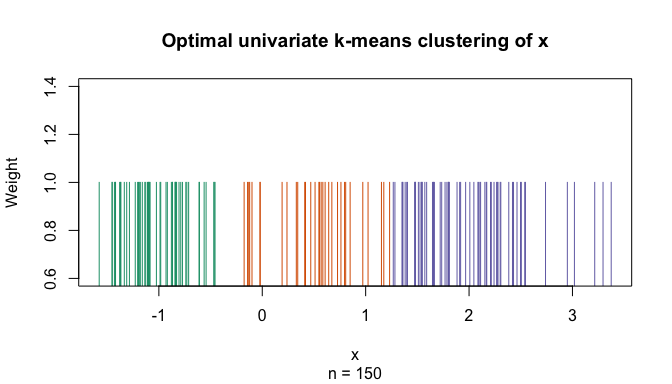plot(x, col=colors[result$cluster], pch=result$cluster, cex=1.5,
main="Optimal univariate clustering with k estimated",
sub=paste("Number of clusters is estimated to be", k))
abline(h=result$centers, col=colors, lty="dashed", lwd=2) legend("topleft", paste("Cluster", 1:k), col=colors, pch=1:k, cex=1, bty="n")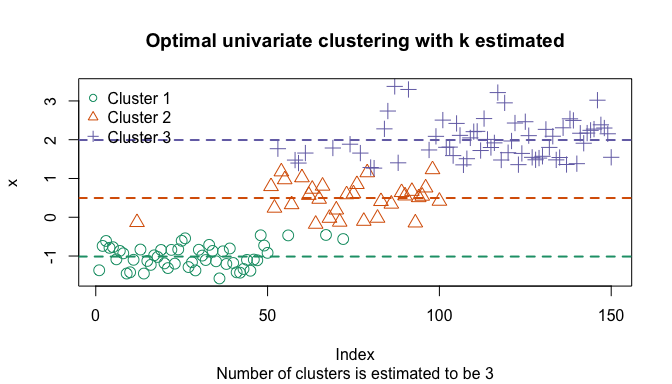## 3. Optimal weighted univariate clustering for time series analysis ### Example 3. We segment a time course to identify peaks using weighted clustering. The input data is the time stamp of obtaining each intensity measurement; the weight is the signal intensity. n <- 160 t <- seq(0, 2*pi*2, length=n) n1 <- 1:(n/2) n2 <- (max(n1)+1):n y1 <- abs(sin(1.5*t[n1]) + 0.1*rnorm(length(n1))) y2 <- abs(sin(0.5*t[n2]) + 0.1*rnorm(length(n2))) y <- c(y1, y2) w <- y^8 # stress the peaks res <- Ckmeans.1d.dp(t, k=c(1:10), w) k <- max(res$cluster)
colors <- brewer.pal(k, "Set1")
plot(res, col.clusters = colors)
grid()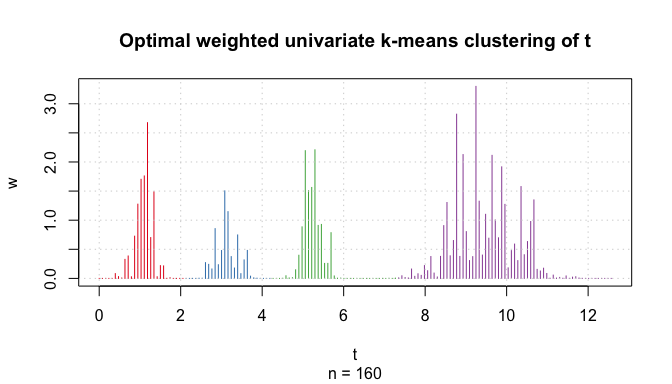plot(t, w, main = "Time course clustering / peak calling",
col=colors[res$cluster], pch=res$cluster, type="h",
xlab="Time t", ylab="Transformed intensity w")
grid()
abline(v=res$centers, col="gray", lty="dashed") text(res$centers, max(w) * .95, cex=0.75, font=2,
paste(round(res$size / sum(res$size) * 100), "/ 100"))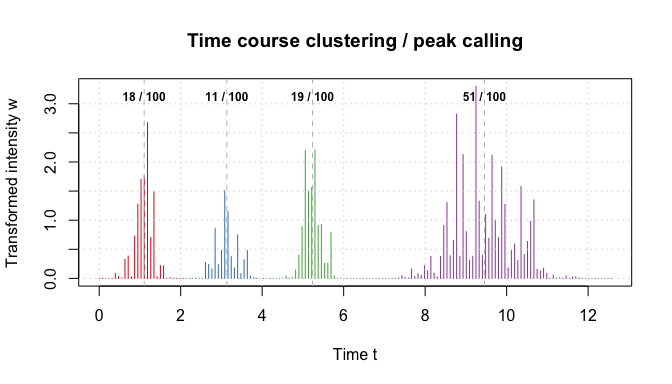## 4. Obtaining midpoints between consecutive clusters

It is often desirable to visualize boundaries between consecutive clusters. The ahist() function offers several ways to estimate cluster boundaries. The simplest is to use the midpoint between the two closest points in two consecutive clusters, as illustrated in the code below.

x <- c(7, 4, 1, 8, 15, 22, -1)
k <- 3
ckm <- Ckmeans.1d.dp(x, k=k)
midpoints <- ahist(ckm, style="midpoints", data=x, plot=FALSE)\$breaks[2:k]

colors <- brewer.pal(k, "Set2")
plot(ckm, col.clusters = colors, lwd=5,
main="Midpoints as cluster boundaries")
abline(v=midpoints, col="RoyalBlue", lwd=3, lty=2)
legend("topright", "Midpoints", lwd=3, lty=2, col="RoyalBlue")